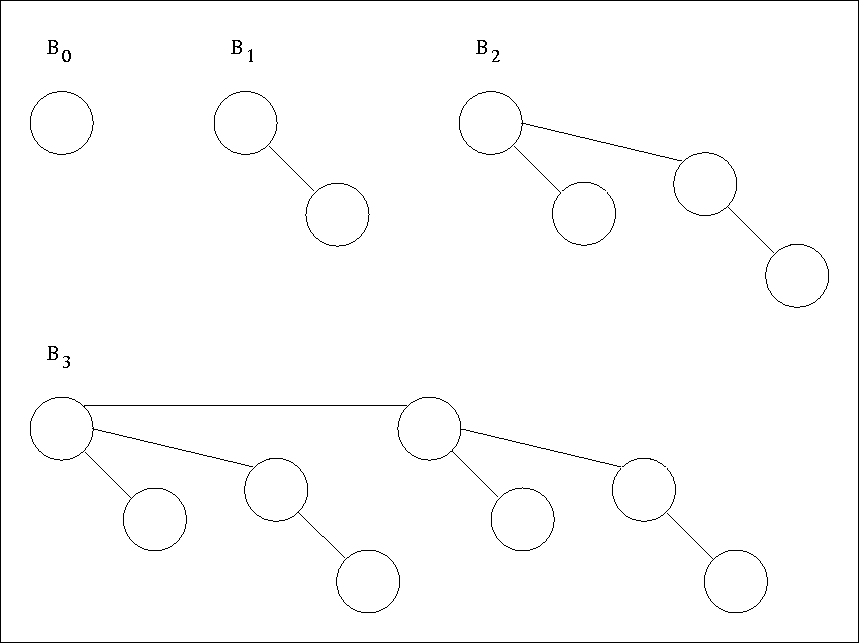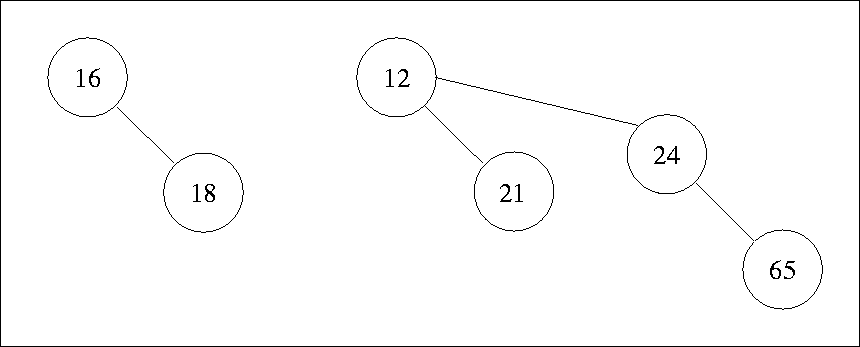Next: 6.2.1 Binomial Queue Operations Up: 6. Priority Queues Previous: 6.1.2 Creating Heap

# 6.2 Binomial Queues

REF.
Jean Vuillemin. A data structure for manipulating priority queues. Communications of the ACM, Volume 21, Number 4, pp.309-315, 1978.
REF.
Mark R. Brown. Implementation and analysis of binomial queue algorithms. SIAM Journal on Computing, Volume 7, Number 3, pp. 298-319, 1978.
• Support merge, insert, and deletemin operations in O(log n) worstcase time.
• A binomial queue is a forest of heap-ordered trees.
• Each of the heap-ordered trees is of a constrained form known as a binomial tree.• A binomial tree of height 0 is a one-node tree; A binomial tree Bk of height k is formed by attaching a binomial tree Bk - 1 to the root of another binomial tree, Bk - 1.
• See Figure 6.5 for an example of binomial tree.
• A binomial tree Bk consists of a root with children B0, B1, ... Bk - 1.
• Bk has exactly 2k nodes.
• Number of nodes at depth d is kCd
• If we impose heap order on the binomial trees and allow at most one binomial tree of any height, we can uniquely represent a priority queue of any size by a forest of binomial trees.
• Example: A priority queue of size 13 could be represented by the forest B3, B2, B0. A natural way of writing this is: 1101. See Figure 6.6 for a binomial queue with six elements.Next: 6.2.1 Binomial Queue Operations Up: 6. Priority Queues Previous: 6.1.2 Creating Heap
eEL,CSA_Dept,IISc,Bangalore